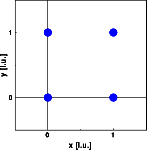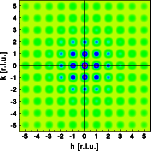Interactive Tutorial about Diffraction Reciprocal space II: Square latticeReciprocal space Reciprocity Square lattice Rectangular lattice Oblique lattice Translation of lattice Rotation lattice Interactive examples 2D crystal builder Planes and HKL's Finite size effect Goto Contents These next three examples illustrate the correlation between real space lengths and angles and their counterparts in reciprocal space. This relationship can also be explored using the interactive crystal builder. To begin with, lets simulate a simple square lattice. Two by two unit cells are simulated, each containing one atom at (0 0). In 3D this would be a cubic lattice. This miniature crystal is sufficient in size to illustrate the geometrical relationships. By increasing the size, we will just decrease the FWHM of the Bragg reflections. The pictures below show the atoms and the corresponding Fourier transform. Click on the pictures to get a full screen version.The reciprocal space geometry corresponds to that of the real space. The reciprocal base vectors are of equal length and orthogonal to each other. © Th. Proffen and R.B. Neder, 2003